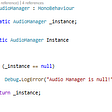# What is Dynamic Programming?

(this was originally sent to the BaseClass newsletter: computing topics explained in plain English)

# Why should I care?

Dynamic programming is the process of breaking down a larger problem into smaller problems.

By using the answers to those smaller problems, we can find the overall solution more efficiently.

We’ll also learn about the term ‘memoization’, and how it relates to dynamic programming.

# How does it work?

The usual example of dynamic programming is the Fibonacci Sequence. It’s a good example, so we’ll use it here too.

If you’re already familiar with the Fibonacci Sequence (and calculating it using recursion), then feel free to skip this next section.

If you’re not sure what that means, or you want a quick refresher, read on…

## The Fibonacci Sequence

This is a sequence of numbers, where each number is calculated by adding the previous two together:

Imagine I ask you to programmatically calculate the 6th number in the sequence (which is `8`, as shown above).

How would you calculate it?

Here’s some JavaScript code for a function that does just that:

`function f(n) {    // The first and second values are always 1    if (n == 1 || n == 2)        return 1;    // Calculate the previous two values in the sequence    // using this same function    return f(n - 1) + f(n - 2)}// Calculate the 6th value in the sequencelet answer = f(6)`

This will work fine, but it’s slow.

You can see that the function calls itself; it is recursive.

To calculate the 6th Fibonacci number, we first need to calculate the 4th and 5th Fibonacci numbers.

Each of those then have to calculate their previous numbers, and this repeats all the way down to the beginning of the sequence.

Here’s what that looks like if we draw it out as a graph.

You can see that there’s a lot of duplication here. For example, the 2nd value in the sequence is calculated 5 times!

For small numbers in the sequence, this isn’t too bad, but it quickly gets worse. For later numbers in the sequence, this would soon become impractical, even on a modern computer.

So how do we do better?

## Dynamic programming and memoization

One way we could improve this function is to store the results of our previous calculations as we go along.

That way, we only need to calculate each number in the Fibonacci sequence once.

This is the essence of dynamic programming:

Dynamic programming is breaking the larger problem into smaller problems, and using those to get to the answer.

Because we’re achieving this by storing the previous results for later, we’re also using ‘Memoization’:

Memoization is storing the result of a previous calculation so that we can use it later, to make the overall algorithm more efficient.

We could implement these concepts on the recursive approach above, but it’s easier to follow if we use a ‘bottom up’ approach.

Let’s look at the code first, and then we can discuss why this is called a ‘bottom up’ algorithm:

`function f(n) {    // The first and second values are always 1    if (n == 1 || n == 2)        return 1    let result = 0    // Used to store the previous two results    let lastButOneValue = 1    let lastValue = 1    // Work from item 3 of the sequence (items 1 and 2 are a     // special case, see above), calculate each value in turn    for (let i = 3; i <= n; i++) {        // Calculate this number by adding together the         // previous two        result = lastValue + lastButOneValue        // Update the values of the         // previous two items in the sequence        lastButOneValue = lastValue        lastValue = result    }    return result}// Calculate the 6th value in the sequencelet answer = f(6)`

Here, we calculate the Fibonacci sequence in order — all the way from the beginning — until we get to the number in the sequence that we need.

As we go along, we store the results of the previous value, and the one before that.

Getting the next value is then trivial.. just add those two together.

Here’s the graph from the original (inefficient) algorithm again, but we’ve highlighted only the calculations we’re making in this new version:

You can see that this time, rather than starting from the answer and working backwards, we work forwards until we reach the value we need.

When we visualise it, we’re working from the bottom of the graph upwards — hence a ‘bottom up’ approach.

This algorithm is much more efficient, there’s no duplication at all!

We learned some new terms here, so let’s recap:

## Recap

Our latest algorithm uses dynamic programming, because we’re breaking the bigger problem into smaller problems, and using their results to get to the overall answer.

It also uses memoization, because we’re storing the results of the previous step to avoid re-calculating them again later.

It was a ‘bottom up’ approach, because when we visualize the problem as a graph, we’re working from the base of the graph upwards (rather than top-down, as in the less efficient algorithm).

# Want to know more?

--

--

--

## More from Dave Saunders

Developer. Lapsed blogger. 10 years of half-finished git repos.

Love podcasts or audiobooks? Learn on the go with our new app.

## Singleton: Audio Manager## Intertect Journal: Day 1## Improve Product Demo Meetings with these Eight Actionable Tips## New Google Developers Profile — Claim yours now## The ultimate CSS battle: Grid vs Flexbox## Attack while jumping## Introduction to android## Dave Saunders

Developer. Lapsed blogger. 10 years of half-finished git repos.

## Recursion forever## Unlucky Guesses## 2 Factors Slowing Down Your Code Debugging and How To Improve## What is Recursion ? What is Recursion ? What is Recursion ?